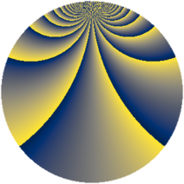# Properties

 Label 1014.2.qLevel $1014$ Weight $2$ Character orbit 1014.q Rep. character $\chi_{1014}(55,\cdot)$ Character field $\Q(\zeta_{39})$ Dimension $720$ Sturm bound $364$

# Related objects

## Defining parameters

 Level: $$N$$ $$=$$ $$1014 = 2 \cdot 3 \cdot 13^{2}$$ Weight: $$k$$ $$=$$ $$2$$ Character orbit: $$[\chi]$$ $$=$$ 1014.q (of order $$39$$ and degree $$24$$) Character conductor: $$\operatorname{cond}(\chi)$$ $$=$$ $$169$$ Character field: $$\Q(\zeta_{39})$$ Sturm bound: $$364$$

## Dimensions

The following table gives the dimensions of various subspaces of $$M_{2}(1014, [\chi])$$.

Total New Old
Modular forms 4464 720 3744
Cusp forms 4272 720 3552
Eisenstein series 192 0 192

## Trace form

 $$720q - 2q^{2} + 30q^{4} - 4q^{5} + 4q^{8} + 30q^{9} + O(q^{10})$$ $$720q - 2q^{2} + 30q^{4} - 4q^{5} + 4q^{8} + 30q^{9} - 2q^{10} + 8q^{11} + 2q^{13} - 8q^{14} + 4q^{15} + 30q^{16} + 6q^{17} + 4q^{18} + 2q^{20} - 8q^{21} + 48q^{22} + 8q^{23} - 72q^{25} - 2q^{26} + 6q^{29} - 4q^{30} + 68q^{31} - 2q^{32} + 4q^{33} + 4q^{34} + 16q^{35} + 30q^{36} - 18q^{37} - 8q^{38} - 12q^{39} + 30q^{40} + 2q^{41} - 4q^{42} + 8q^{43} - 16q^{44} + 2q^{45} - 16q^{47} + 86q^{49} - 4q^{52} + 102q^{53} + 128q^{55} + 4q^{56} - 8q^{57} + 58q^{58} - 8q^{59} + 44q^{60} - 6q^{61} + 12q^{62} - 60q^{64} + 26q^{65} + 8q^{66} + 148q^{67} + 6q^{68} - 4q^{69} + 224q^{70} - 320q^{71} - 2q^{72} + 52q^{73} + 200q^{74} + 8q^{75} + 52q^{76} - 32q^{77} - 24q^{79} + 2q^{80} + 30q^{81} + 2q^{82} + 4q^{84} + 116q^{85} + 156q^{86} + 68q^{87} - 4q^{88} + 20q^{89} + 4q^{90} + 104q^{91} - 16q^{92} + 156q^{93} + 52q^{94} - 392q^{95} + 168q^{97} + 6q^{98} - 16q^{99} + O(q^{100})$$

## Decomposition of $$S_{2}^{\mathrm{new}}(1014, [\chi])$$ into newform subspaces

The newforms in this space have not yet been added to the LMFDB.

## Decomposition of $$S_{2}^{\mathrm{old}}(1014, [\chi])$$ into lower level spaces

$$S_{2}^{\mathrm{old}}(1014, [\chi]) \cong$$ $$S_{2}^{\mathrm{new}}(169, [\chi])$$$$^{\oplus 4}$$$$\oplus$$$$S_{2}^{\mathrm{new}}(338, [\chi])$$$$^{\oplus 2}$$$$\oplus$$$$S_{2}^{\mathrm{new}}(507, [\chi])$$$$^{\oplus 2}$$